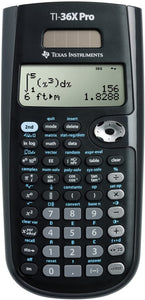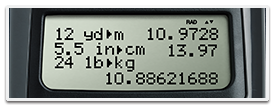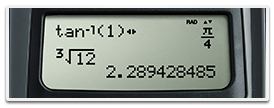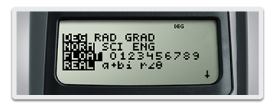# TI-36X Pro

Regular price
HKD200.00
Sale price
HKD200.00
Regular price
Sold out
Unit price
per

### TI-36X Pro scientific calculator

Advanced, four-line scientific calculator, with higher-level math and science functionality. Ideal for computer science and engineering courses in which graphing technology may not be permitted.

Key features

• Four-line display
• One- and two-variable statistics
• MultiView™ display shows multiple calculations at the same time on screen
• Select degrees/radians, floating/fix, number format modes
• Choose from three solvers: numeric equation, polynomial and system of linear equations
• Display a defined function in a tabular form
• Determine the numeric derivative and integral for real functions
• Perform vectors and matrices using a vector and matrix entry window

Ideal for:

• Algebra I and II
• Geometry
• Trigonometry
• Statistics
• Calculus
• Biology
• Chemistry
• Physics
• Computer science
• College math
• College science
• College engineering

View multiple calculations at the same time
Compare results and explore patterns on-screen with the MultiView™ display, which supports up to four lines.See math exactly as it appears in textbooks
See math expressions, symbols and stacked fractions exactly the way they appear in textbooks — no need to adapt to a technical syntax; also provides quick access to frequently used functions.Built-in solvers
Intuitive prompts allow one to easily solve numeric equation, polynomial and system of linear equations.Explore (x,y) table of values
Students can easily explore an (x,y) table of values for a given function, automatically or by entering specific x values.Exam acceptance

The TI-36X Pro scientific calculator is approved for use on SAT®, ACT®, and AP® exams.

SAT® and AP® are trademarks registered by the College Board. ACT is a registered trademark of ACT, Inc. Neither are affiliated with, nor endorse, TI products. Policies subject to change. Visit www.collegeboard.org and www.act.org.